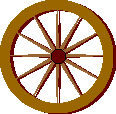Calculate a unit normal to (-5, 3)T.

(3, 5)/(34). Or you might pick the other one (there are two): (-3, -5)/(34).

# Infinitely Many NormalsVectors in three dimensions, also, have unit normals. But a unit normal vector to a given vector is not unique. Think of the axle of a wagon wheel. All the spokes are orthogonal to the axle. Any line in the plane of the spokes is orthogonal to the axle; and there are an infinite number of them.

### QUESTION 11:

Find several normals to the vector represented by (1, 2, 2)T.